Question

# An analytical chemist is titrating 182.1 mL. of a 0.4900 M solution of acetic acid (HCH,CO,)...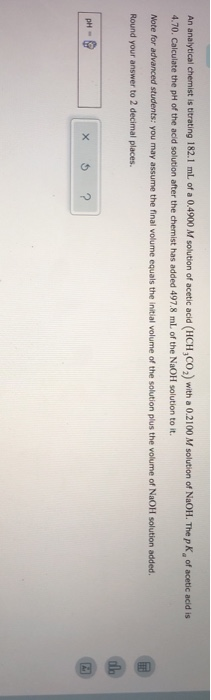An analytical chemist is titrating 182.1 mL. of a 0.4900 M solution of acetic acid (HCH,CO,) with a 0.2100 M solution of NaOH. The p K of acetic acid is 4.70. Calculate the pH of the acid solution after the chemist has added 497.8 ml of the NaOH solution to it. Note for advanced students: you may assume the final volume equals the initial volume of the solution plus the volume of NaOH solution added. Round your answer to 2 decimal places. pH-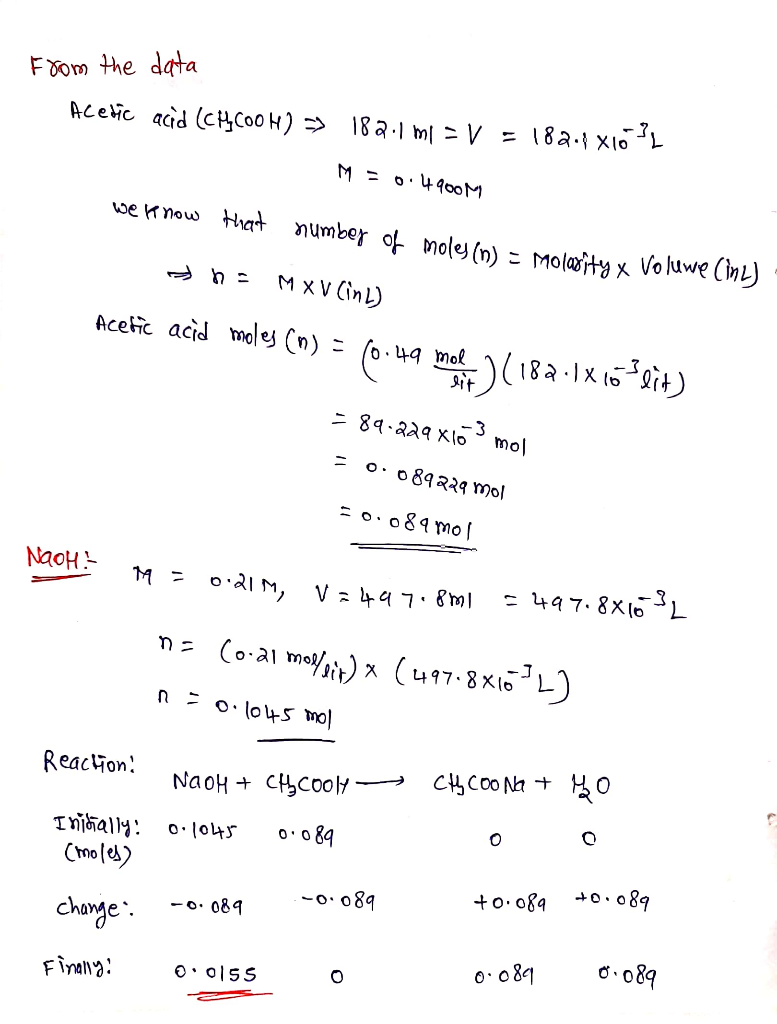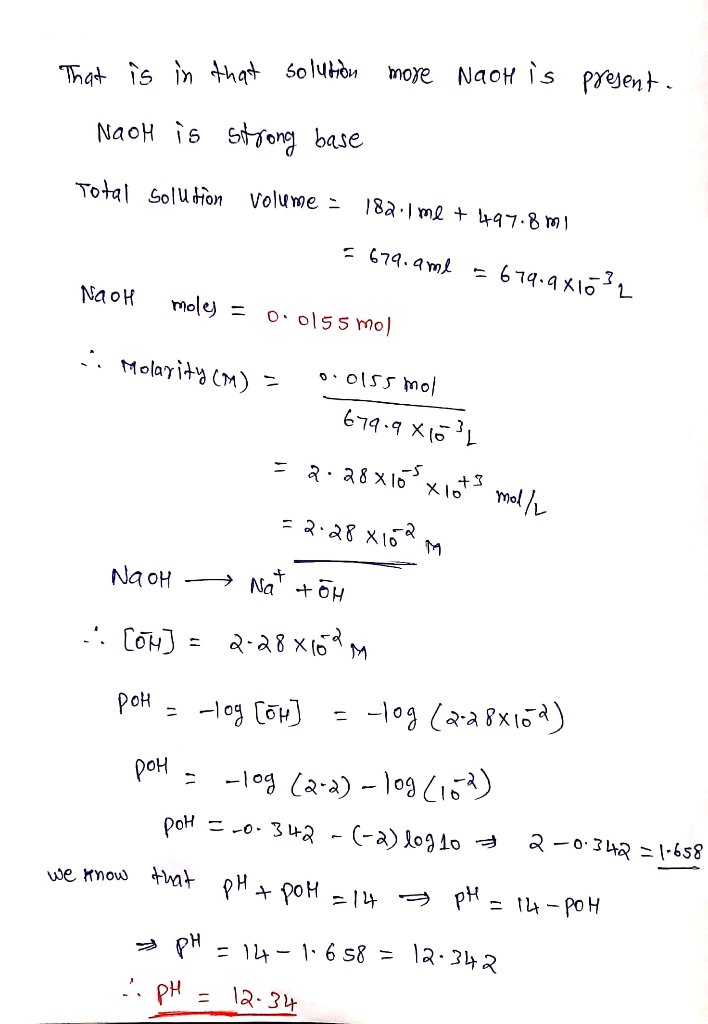#### Earn Coins

Coins can be redeemed for fabulous gifts.

Similar Homework Help Questions
• ### An analytical chemist is titrating 106,3 ml. of a 0.9500 M solution of acetic acid (HCH,CO,)...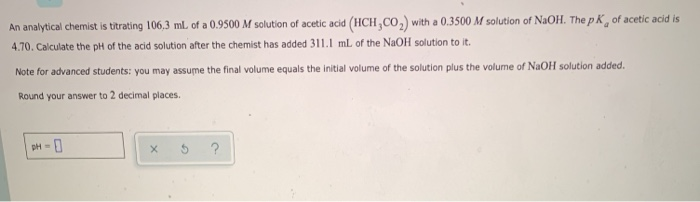An analytical chemist is titrating 106,3 ml. of a 0.9500 M solution of acetic acid (HCH,CO,) with a 0.3500 M solution of NaOH. The p K, of acetic acid is 4.70. Calculate the pH of the acid solution after the chemist has added 311.1 mL of the NaOH solution to it. Note for advanced students: you may assume the final volume equals the initial volume of the solution plus the volume of NaOH solution added. Round your answer to 2...

• ### An analytical chemist is titrating 54.4 ml of a 0.9400 M solution of acetic acid (HCH,CO,)...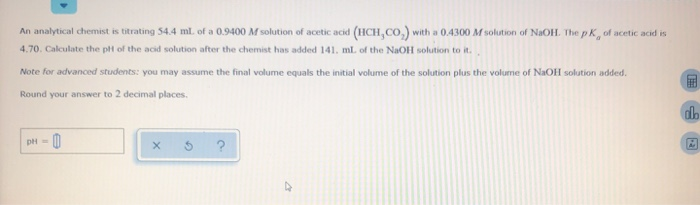An analytical chemist is titrating 54.4 ml of a 0.9400 M solution of acetic acid (HCH,CO,) with a 0.4300 M solution of NaOH. The pk of acetic acid is 4.70. Calculate the pit of the acid solution after the chemist has added 141 ml of the NaOH solution to it. Note for advanced students: you may assume the final volume equals the initial volume of the solution plus the volume of NaOH solution added. Round your answer to 2 decimal...

• ### An analytical chemist is titrating 69.7 mL of a 0.3900 M solution of acetic acid (HCH,...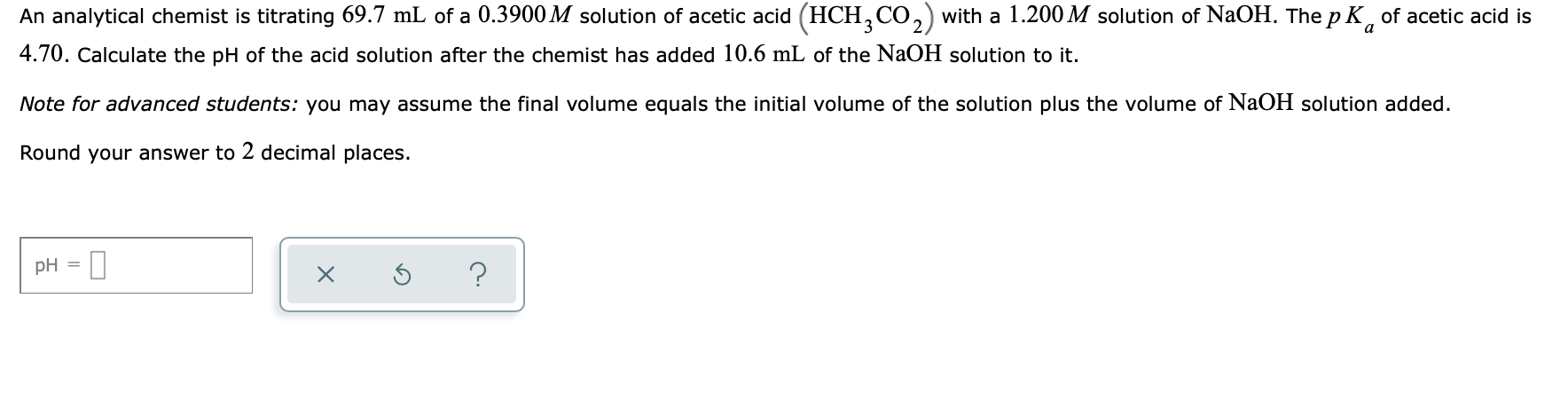An analytical chemist is titrating 69.7 mL of a 0.3900 M solution of acetic acid (HCH, CO,) with a 1.200 M solution of NaOH. The pK, of acetic acid is 4.70. Calculate the pH of the acid solution after the chemist has added 10.6 mL of the NaOH solution to it. Note for advanced students: you may assume the final volume equals the initial volume of the solution plus the volume of NaOH solution added. Round your answer to 2...

• ### An analytical chemist is titrating 105.5 mL of a 0.4700 M solution of acetic acid (HCH,CO)...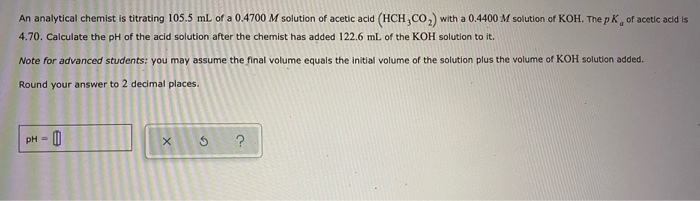An analytical chemist is titrating 105.5 mL of a 0.4700 M solution of acetic acid (HCH,CO) with a 0.4400 M solution of KOH. The pKof acetic acid is 4.70. Calculate the pH of the acid solution after the chemist has added 122.6 mL of the KOH solution to it. Note for advanced students: you may assume the final volume equals the initial volume of the solution plus the volume of KOH solution added. Round your answer to 2 decimal places....

• ### An analytical chemist is titrating 72.8 ml. of a 0.6300 M solution of acetic acid (HCH,CO,) with a 0.6600 M solutio...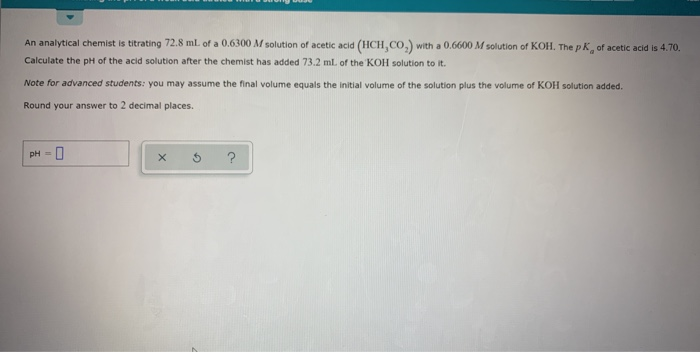An analytical chemist is titrating 72.8 ml. of a 0.6300 M solution of acetic acid (HCH,CO,) with a 0.6600 M solution of KOH. The pk, of acetic acid is 4.70. Calculate the pH of the acid solution after the chemist has added 73.2 ml of the KOH solution to it Note for advanced students: you may assume the final volume equals the initial volume of the solution plus the volume of KOH solution added. Round your answer to 2 decimal...

• ### An analytical chemist is titrating 132.2 mL of a 0.9700 M solution of acetic acid (HCH,CO2)...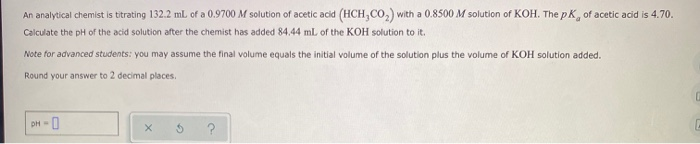An analytical chemist is titrating 132.2 mL of a 0.9700 M solution of acetic acid (HCH,CO2) with a 0.8500 M solution of KOH. The pk of acetic acid is 4.70. Calculate the pH of the acid solution after the chemist has added 84.44 mL of the KOH solution to it. Note for advanced students: you may assume the final volume equals the initial volume of the solution plus the volume of KOH solution added. Round your answer to 2 decimal...

• ### An analytical chemist is titrating 160.3mL of a 1.100M solution of acetic acid HCH3CO2 with a...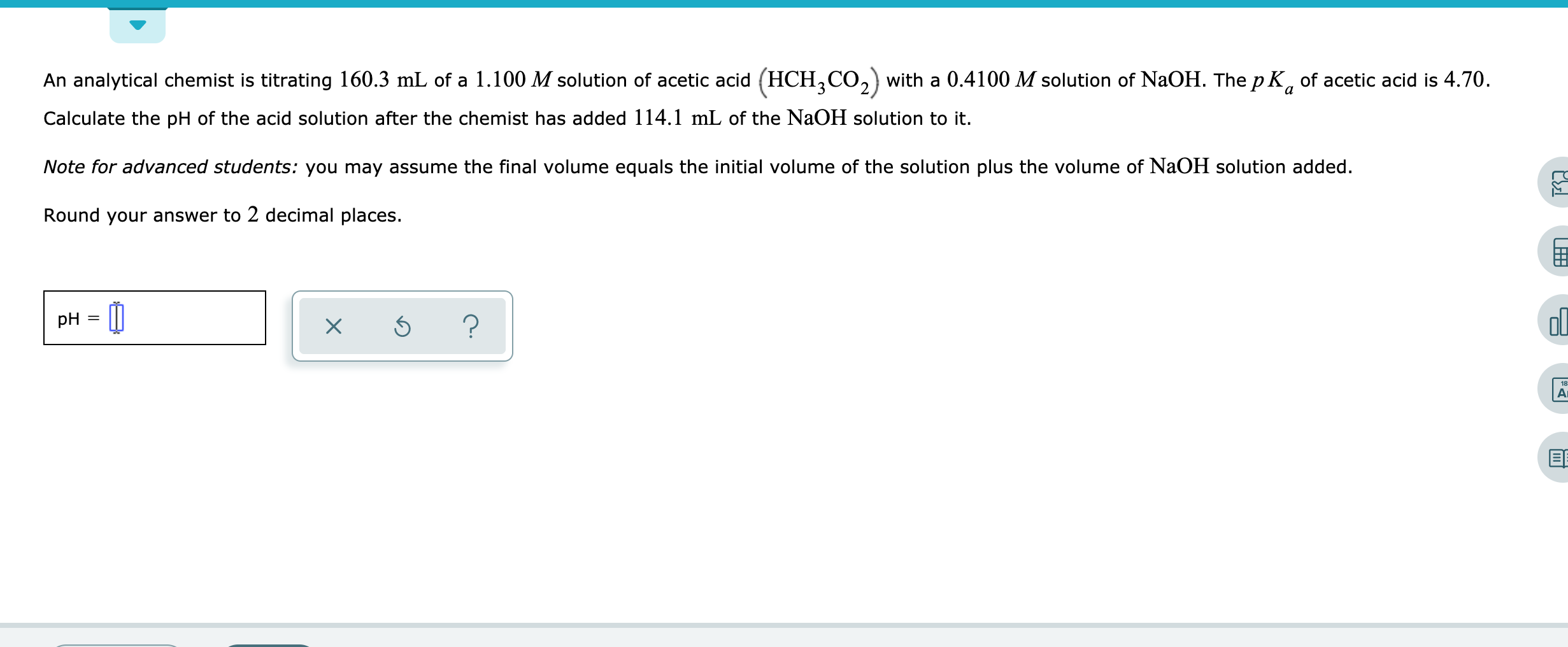An analytical chemist is titrating 160.3mL of a 1.100M solution of acetic acid HCH3CO2 with a 0.4100M solution of NaOH. The pKa of acetic acid is 4.70. Calculate the pH of the acid solution after the chemist has added 114.1mL of the NaOH solution to it. Note for advanced students: you may assume the final volume equals the initial volume of the solution plus the volume of NaOH solution added. Round your answer to 2 decimal places. An analytical chemist...

• ### An analytical chemist is titrating 194.8 mL of a 0.8600 M solution of acetic acid (HCH3CO2)...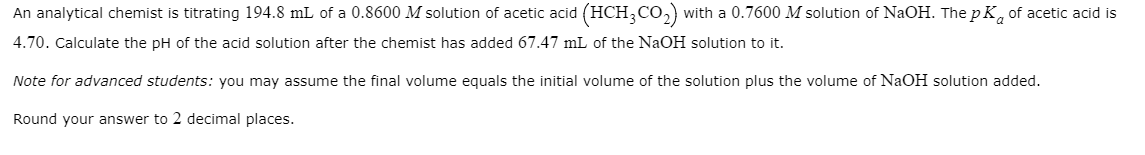An analytical chemist is titrating 194.8 mL of a 0.8600 M solution of acetic acid (HCH3CO2) with a 0.7600 M solution of NaOH. The pKof acetic acid is 4.70. Calculate the pH of the acid solution after the chemist has added 67.47 mL of the NaOH solution to it. Note for advanced students: you may assume the final volume equals the initial volume of the solution plus the volume of NaOH solution added. Round your answer to 2 decimal places.

• ### An analytical chemist is titrating 66.3 mL of a 0.9100 M solution of benzoic acid (HCH,CO2)...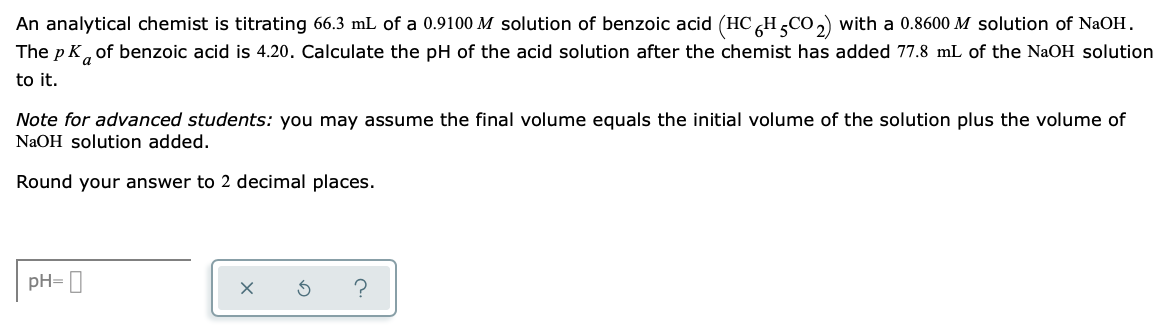An analytical chemist is titrating 66.3 mL of a 0.9100 M solution of benzoic acid (HCH,CO2) with a 0.8600 M solution of NaOH. The pK, of benzoic acid is 4.20. Calculate the pH of the acid solution after the chemist has added 77.8 mL of the NaOH solution to it. Note for advanced students: you may assume the final volume equals the initial volume of the solution plus the volume of NaOH solution added. Round your answer to 2 decimal...

• ### An analytical chemist is titrating 238.8 mL of a 1.200 M solution of benzoic acid (HCH...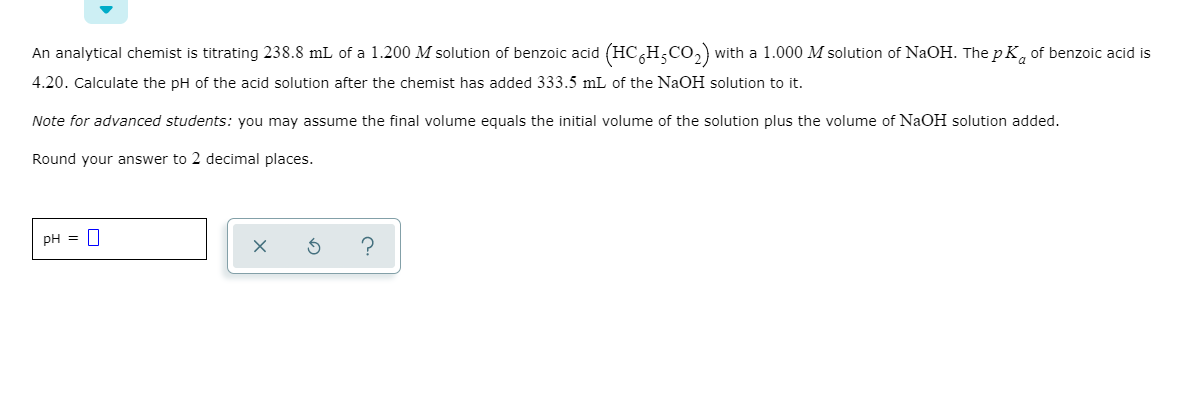An analytical chemist is titrating 238.8 mL of a 1.200 M solution of benzoic acid (HCH CO2) with a 1.000 M solution of NaOH. The pk, of benzoic acid is 4.20. Calculate the pH of the acid solution after the chemist has added 333.5 mL of the NaOH solution to it. Note for advanced students: you may assume the final volume equals the initial volume of the solution plus the volume of NaOH solution added. Round your answer to 2...